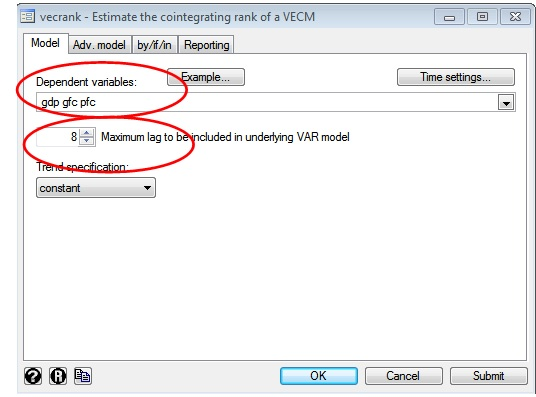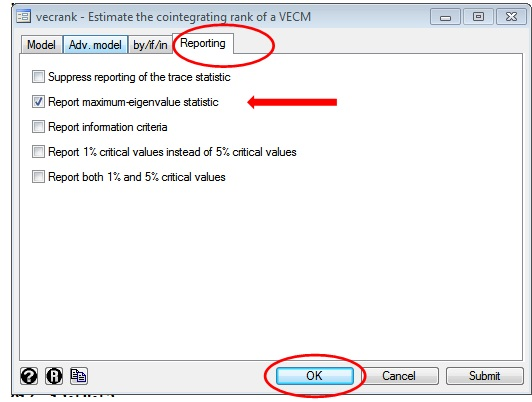# How to perform Johansen cointegration test in VAR with three variables?

The previous article showed lag selection and stationarity for Vector Auto Regression (VAR) with three variables; Gross Domestic Product (GDP), Gross Fixed Capital Formation (GFC) and Private Final Consumption (PFC). This article shows the co-integration test for VAR with three variables. To perform the Johansen cointegration test, follow the below steps.

1. Click on ‘Statistics’ on Result window
2. Select ‘Multivariate Time-series’
3. Select ‘Co-integrating rank of a VECM’

The ‘vecrank’ dialogue box will appear. Select values for two options ‘Dependent variables’ and ‘Maximum lags to be included in underlining VAR Model’.Figure 2: Vecrank window on STATA for Johansen co-integration analysis in VAR model with three variables

In ‘Dependent variables’, select the three-time series variables GDP, GFC and PFC. The co-integration analysis takes the case of non-stationary variables to check for long-term causality. Therefore take GDP, GFC and PFC instead of their first differences. Next, select the number of lags. In this case, conduct lag selected criteria similar to the previous analysis with the number of lags is 8 as shown in the figure above. Click on ‘OK’.

Click on ‘Reporting’ tab. Select ‘Report maximum-eigenvalue statistic’ as shown in the figure below. Then click on ‘OK’.Figure 3: ‘Reporting’ tab of vecrank window on STATA for Johansen co-integration test in VAR with three variables
Use 5E3D454DA1A7D to save 4000 on 4001 - 6000 words standard order of research paper service.

## Johansen cointegration test results

The results for Johansen cointegration test will appear.

##### Command
`vecrank gdp gfc pfc, trend(constant) lags(8) max`

Interpret the result of Johansen cointegration test in parts. First, converge the focus towards three columns; maximum rank, trace statistics or max statistics and critical values.

## Maximum rank zero

Starting from maximum rank zero, the null and alternative hypotheses are as follows:

• Null hypothesis: There is no cointegration.
• Alternative hypothesis: There is cointegration.

At maximum rank zero, the trace statistic (55.177) exceeds critical values (29.68). Therefore reject the null hypothesis so that the time series variables GDP, PFC and GFC are cointegrated. Similarly, for max statistics, the value 40.7477 exceeds a critical value of 20.97, thus rejecting the null hypothesis. Thus, as per maximum rank zero, GDP, GFC and PFC are cointegrated.

## Maximum rank one

For maximum rank one, the null and alternative hypotheses are as follows:

• Null hypothesis: There is a cointegration of equation 1.
• Alternative hypothesis: There is no cointegration of equation 1.

At maximum rank one, the trace statistic (14.4298) does not exceed critical values (15.41). Therefore accept the null hypothesis that there is cointegration of one equation. Similarly, for max statistics, the value 12.4140 do not exceed a critical value of 14.07, therefore accept the null hypothesis. Thus, as per maximum rank one, GDP, GFC and PFC are cointegrated of one equation. The significance of equation one will be explained in the next article.

## Maximum rank two

For maximum rank two, the null and alternative hypotheses are as follows:

• Null hypothesis: There is a cointegration of equation 2.
• Alternative hypothesis: There is no cointegration of equation 2.